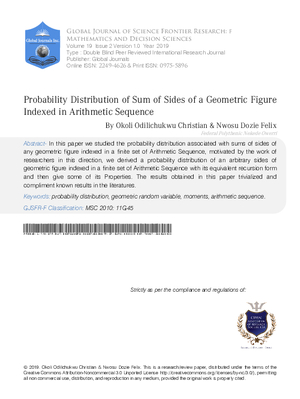### Probability Distribution of Sum of Sides of a Geometric Figure Indexed in Arithmetic Sequence

Authors

• Okoli Odilichukwu Christian

• Nwosu Dozie Felix

Keywords:

probability distribution, geometric random variable, moments, arithmetic sequence

Abstract

In this paper we studied the probability distribution associated with sums of sides of any geometric figure indexed in a finite set of Arithmetic Sequence, motivated by the work of researchers in this direction, we derived a probability distribution of an arbitrary sides of geometric figure indexed in a finite set of Arithmetic Sequence with its equivalent recursion form and then give some of its Properties. The results obtained in this paper trivialized and compliment known results in the literatures.

How to Cite

Okoli Odilichukwu Christian, & Nwosu Dozie Felix. (2019). Probability Distribution of Sum of Sides of a Geometric Figure Indexed in Arithmetic Sequence. Global Journal of Science Frontier Research, 19(F2), 19–38. Retrieved from https://journalofscience.org/index.php/GJSFR/article/view/2473Published

2019-03-15

Issue

Section

Articles